Latest Banking jobs   »

# Quantitative Aptitude Quiz For IDBI AM/Executive 2022- 23rd June

Directions (1-3): The following questions are accompanied by two statements A and B. You have to determine which statements(s) is/are sufficient/necessary to answer the questions.
(a) Statement A alone is sufficient to answer the question, but statement B alone is not sufficient to answer the questions.
(b) Statement B alone is sufficient to answer the question, but statement A alone is not sufficient to answer the question.
(c) Both the statements taken together are necessary to answer the questions, but neither of the statements alone is sufficient to answer the question.
(d) Either statement A or statement B by itself is sufficient to answer the question.

(e) Statements A and B taken together are not sufficient to answer the question.

Q1. Find the sum of digits of given two-digit number?
A. When nine is added to the given two-digit number then the sum of digits of new number formed is 1
B. If given two-digit number is added with the reverse of it than the sum is 110.

Q2. In how much time a boatman can reach a point and come back which is 120km away from his house?
A. He can row 40km upstream in same time in which he can row 80km downstream
B. Time taken by boatman to row 60km upstream is 3.75 hours more than time taken by him to row same distance in downstream.

Q3. ‘A’ and ‘B’ started a business together. ‘B’ is replaced by ‘C’ after 4 months. Find C’s profit after an year?
A. A’s investment is 25% more than B’s investment while ‘B’ invested 6,000 more than ‘C’
B. B’s profit out of total profit is Rs. 16,000.

Directions (4-5): The following questions are accompanied by two statements (A) and (B) . You have to determine which statements(s) is/are sufficient/necessary to answer the questions.

Q4. O is center, find area of shaded region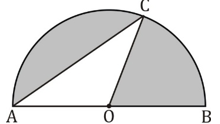(A) Length of AO is given.
(B) AC is given in multiple of radius of semicircle.

(a) Only A
(b) Only B
(c) Either Only A or Only B
(d) A and B together
(e) A and B together are not sufficient

Q5. In a box three type of balls are there, Black, Red and White. If no. of white balls is given then find out the probability of getting one white ball.
(A) Probability of getting one Red ball is given.
(B) Probability of getting one black ball is given.
(a) Only A
(b) Only B
(c) Either Only A or Only B
(d) A and B together
(e) A and B together are not sufficient

Q6. ‘A’, ‘B’ and ‘C’ together can complete a work in 48 days if they work alternatively. ‘A’ is 25% more efficient than ‘B’ who is 33⅓% less efficient than ‘C’.
Quantity I: Difference between days taken by ‘A’ alone and ‘C’ alone to complete the work.
Quantity II: Days in which ‘A’, ‘B’ and ‘C’ together can complete half of the work.
(a) Quantity I > Quantity II
(b) Quantity I < Quantity II
(c) Quantity I ≥ Quantity II
(d) Quantity I ≤ Quantity II
(e) Quantity I = Quantity II or No relation

Q7. Neeraj invested Rs. X in two different schemes ‘A’ and ‘B’ equally. Scheme A offers 10% p.a. at S.I and scheme B offers 20% p.a. at C.I. After 2 years he got total Rs.2560 interest from both the schemes.
Quantity I: Value of ‘X’
Quantity II: Rs. 7200
(a) Quantity I ≥ Quantity II
(b) Quantity I = Quantity II or No relation
(c) Quantity I > Quantity II
(d) Quantity I ≤ Quantity II
(e) Quantity I < Quantity II

Q8. Three partners invested capital in the ratio 2 : 7 : 9. The time period for which each of them invested was in the ratio of the reciprocals of the amount invested..
Quantity I: Profit share of the partner who brought in the highest capital if the profit is Rs. 1080
Quantity II: Profit share of the partner who brought in the lowest capital if the profit is Rs. 1080
(a) Quantity I > Quantity II
(b) Quantity I ≥ Quantity II
(c) Quantity I < Quantity II
(d) Quantity I = Quantity II or No relation
(e) Quantity I ≤ Quantity II

Q9. X started from a point A towards point B. After 2 hours. Y started from B towards A. By the time X travelled one-fifth of the total distance, Y had also travelled the same. Y’s speed is thrice of that of X’s speed.
Quantity I: Difference in time (in hours) taken by X and Y to reach their respective destinations.
Quantity II: 12 hours
(a) Quantity I = Quantity II or No relation
(b) Quantity I ≤ Quantity II
(c) Quantity I ≥ Quantity II
(d) Quantity I < Quantity II
(e) Quantity I > Quantity II

Q10. A vessel contains 2.5 litres of water and 10 litres of milk. 20% of the contents of the vessel are removed. To the remaining contents, x litres of water is added to reverse the ratio of water and milk. Then y litres of milk is added again to reverse the ratio of water and milk.
Quantity I: Value of ‘y’
Quantity II: Value of ‘x’
(a) Quantity I = Quantity II or No relation
(b) Quantity I ≤ Quantity II
(c) Quantity I ≥ Quantity II
(d) Quantity I < Quantity II
(e) Quantity I > Quantity II

Directions (11-15): In the given questions, two quantities are given, one as ‘Quantity I’ and another as ‘Quantity II’. You have to determine relationship between two quantities and choose the appropriate option:

Q11. In a two digit number, digit at unit place exceeds, the digit in its tens place by 2 and the product of the required number with the sum of its digit is equal to 144.
Quantity I: Value of two digit number
Quantity II: 26
(a) Quantity I > Quantity II
(b) Quantity I < Quantity II
(c) Quantity I ≥ Quantity II
(d) Quantity I ≤ Quantity II
(e) Quantity I = Quantity II or No relation

Q12. Quantity I : Days after which A and B meet. A and B set out to meet each other from two places 165 km apart. A travels 15 km the first day, 14 km second day, 13 km the third day and so on, B travels 10 km the first, 12 km the second day, 14 km the third day and so on.
Quantity II: Number of days required to complete the whole work if A, B and C can complete a piece of work in 10, 12 and 15 days respectively. A left the work 5 days before the work was completed and B left 2 days after A had left.
(a) Quantity I > Quantity II
(b) Quantity I < Quantity II
(c) Quantity I ≥ Quantity II
(d) Quantity I ≤ Quantity II
(e) Quantity I = Quantity II or No relation

Q13. Quantity I: Present age of Randy, if 10 years are subtracted from the present age of Randy, then you would get twelve times of the present age of his grandson Sandy and Sandy is 19 years younger to Sundar whose age is 24.
Quantity II: Average age of the remaining persons in the group if average age of group of 14 persons is 27 years and 9 months. Two persons, each 42 years old, left the group.
(a) Quantity I > Quantity II
(b) Quantity I < Quantity II
(c) Quantity I ≥ Quantity II
(d) Quantity I ≤ Quantity II
(e) Quantity I = Quantity II or No relation

Q14. Quantity I: Percentage profit earned by the shopkeeper if at the time of selling and purchasing he uses weights 10% less and 20% more per kilogram respectively and proffesses to all goods at 5% profit.
Quantity II: ‘x’ ; A book was sold for a certain sum and there was a loss of 20%. Had it been sold for Rs 12 more, there would have been a gain of 30%. ‘x’ would be value of profit percent if the book were sold for Rs 4.8 more than what it was sold for.
(a) Quantity I > Quantity II
(b) Quantity I < Quantity II
(c) Quantity I ≥ Quantity II
(d) Quantity I ≤ Quantity II
(e) Quantity I = Quantity II or No relation

Q15. A group consist of 4 couples in which each of the 4 persons have one wife
Quantity I : Number of ways in which they could be arranged in a straight line such that the men and women occupy alternate positions
Quantity II: Eight times the number of ways in which they be seated around circular table such that men and women occupy alternate position.
(a) Quantity I > Quantity II
(b) Quantity I < Quantity II
(c) Quantity I ≥ Quantity II
(d) Quantity I ≤ Quantity II
(e) Quantity I = Quantity II or No relation

Solutions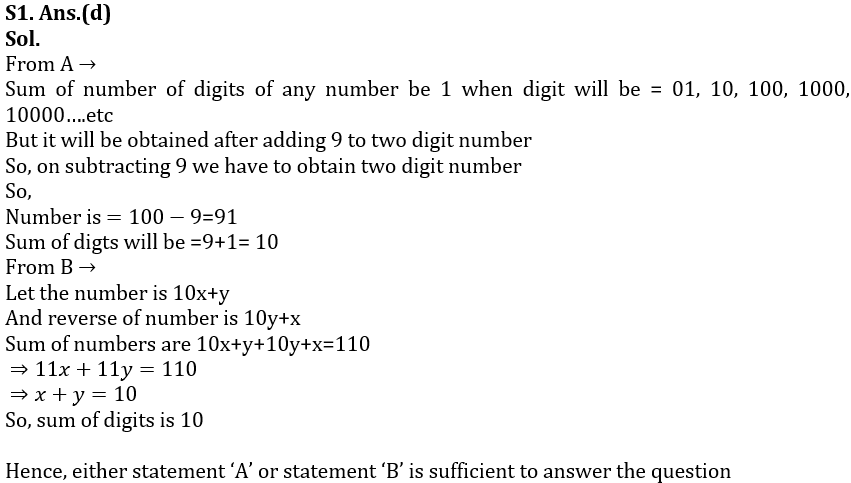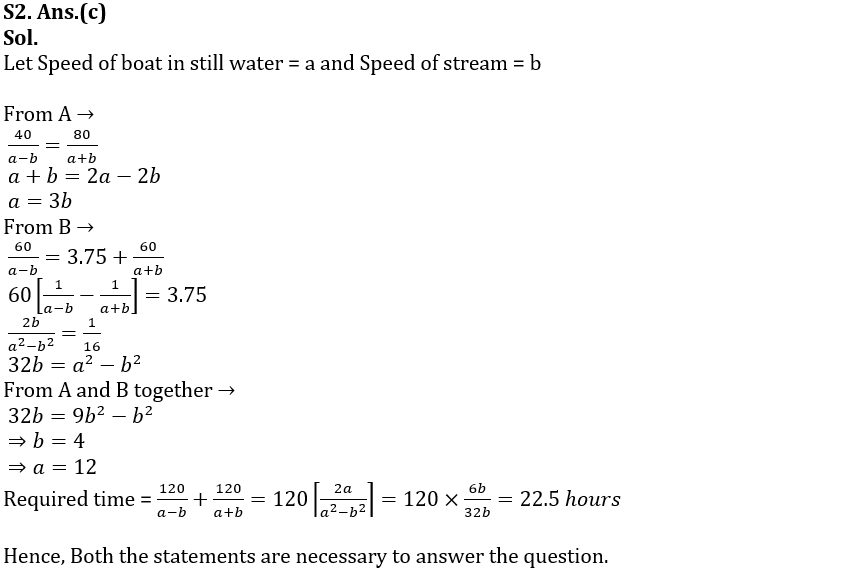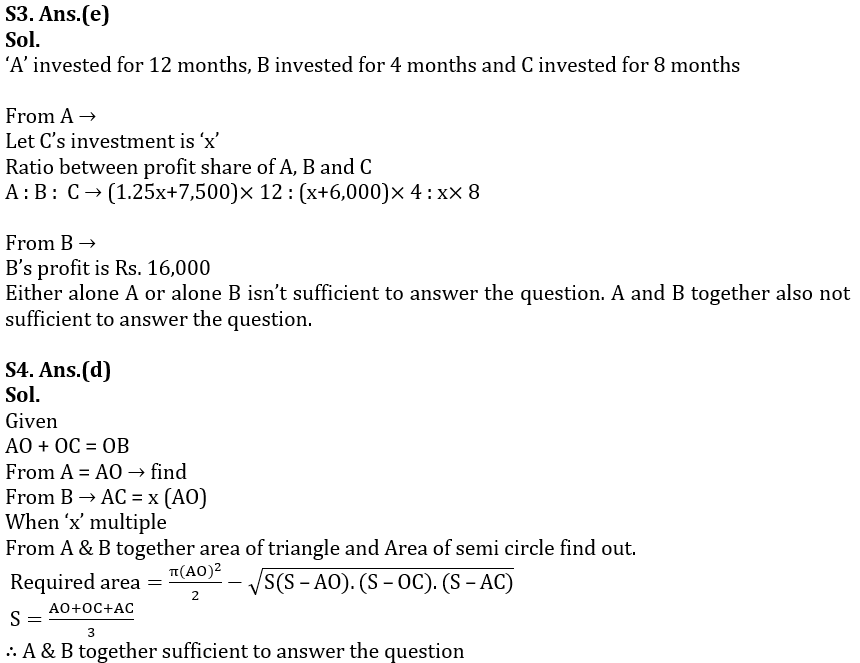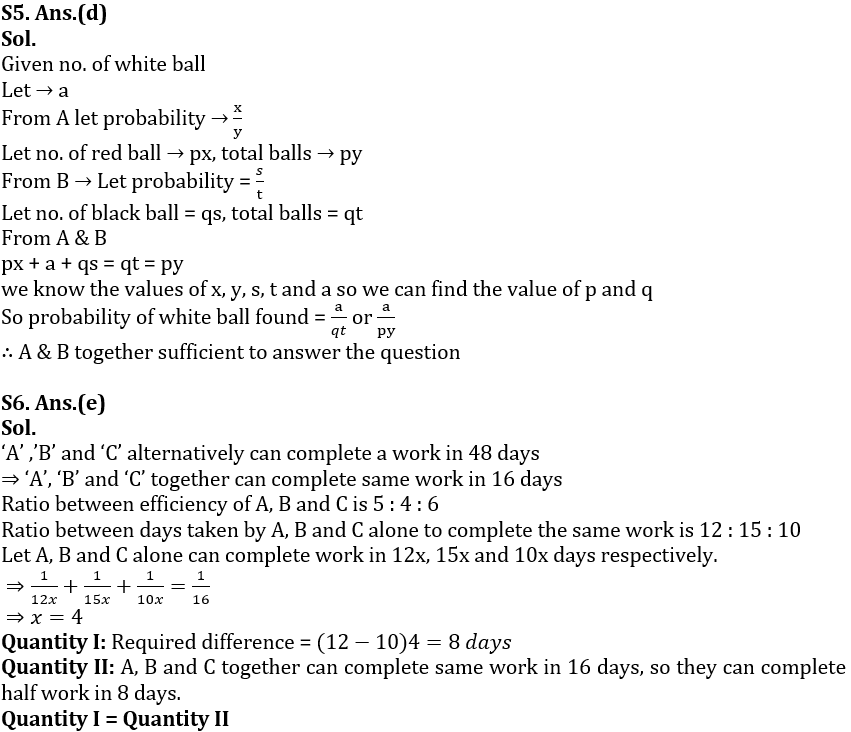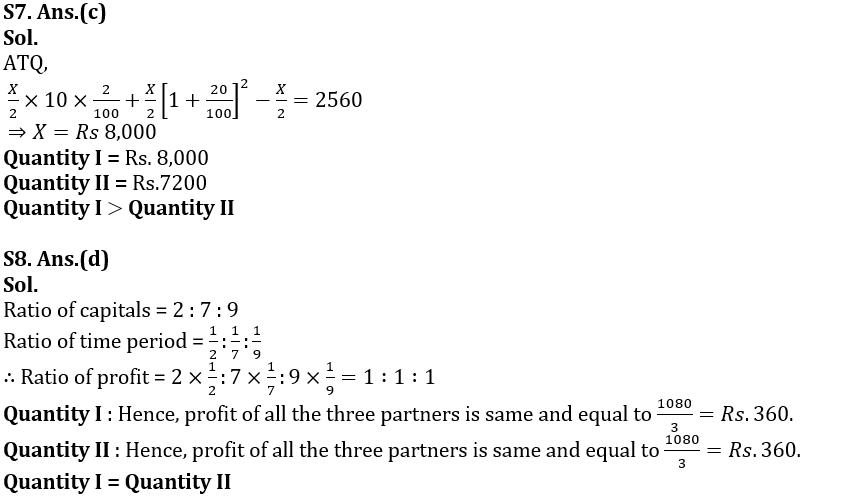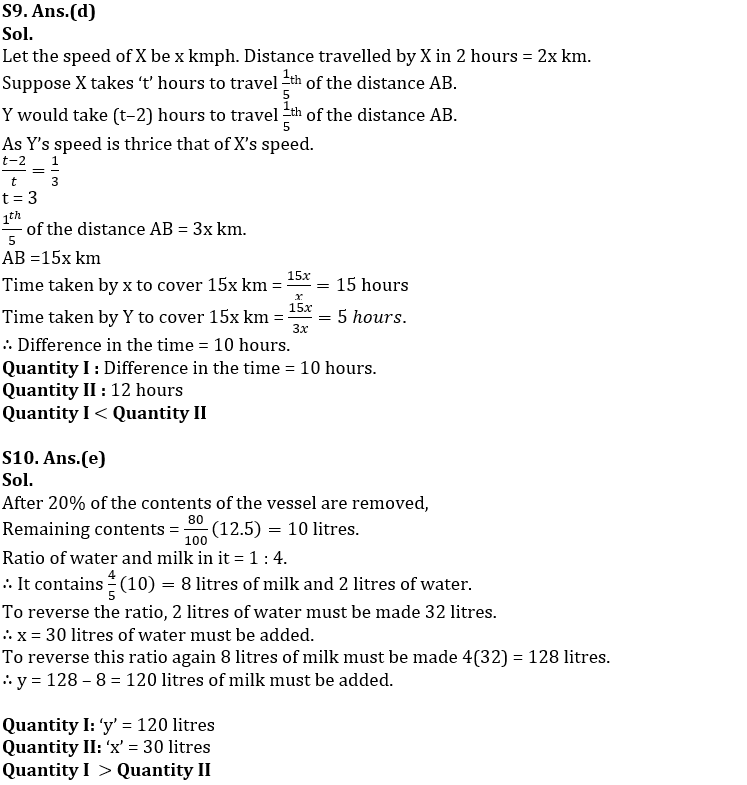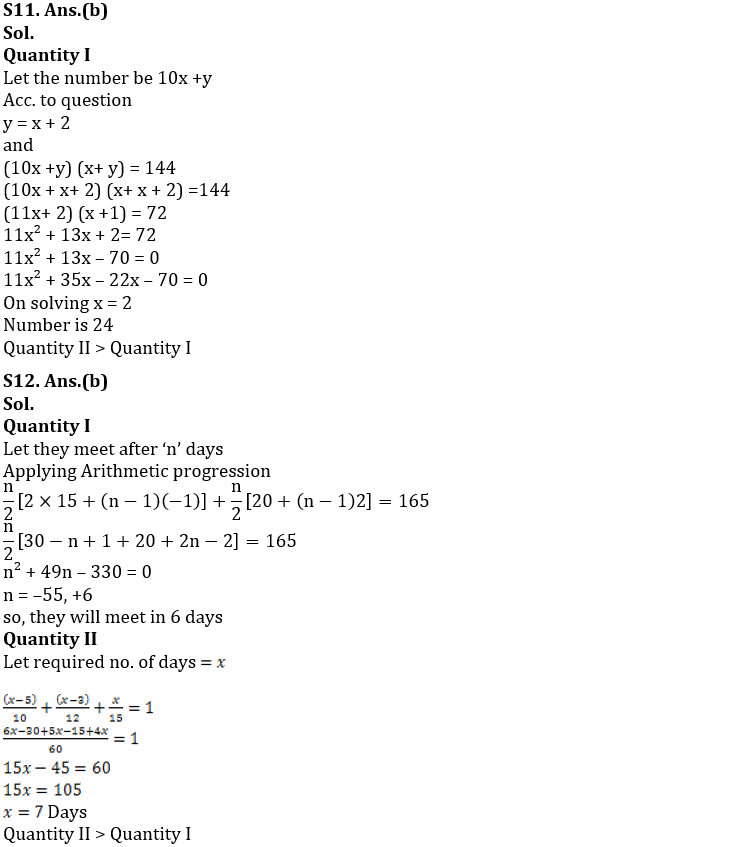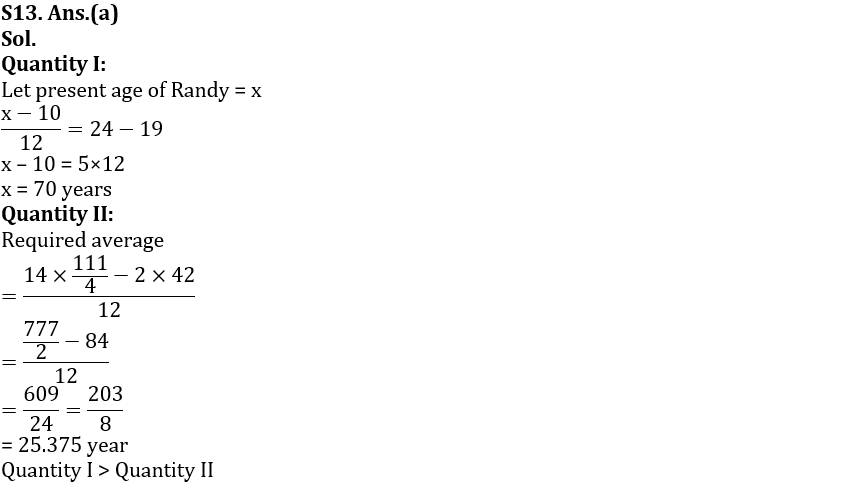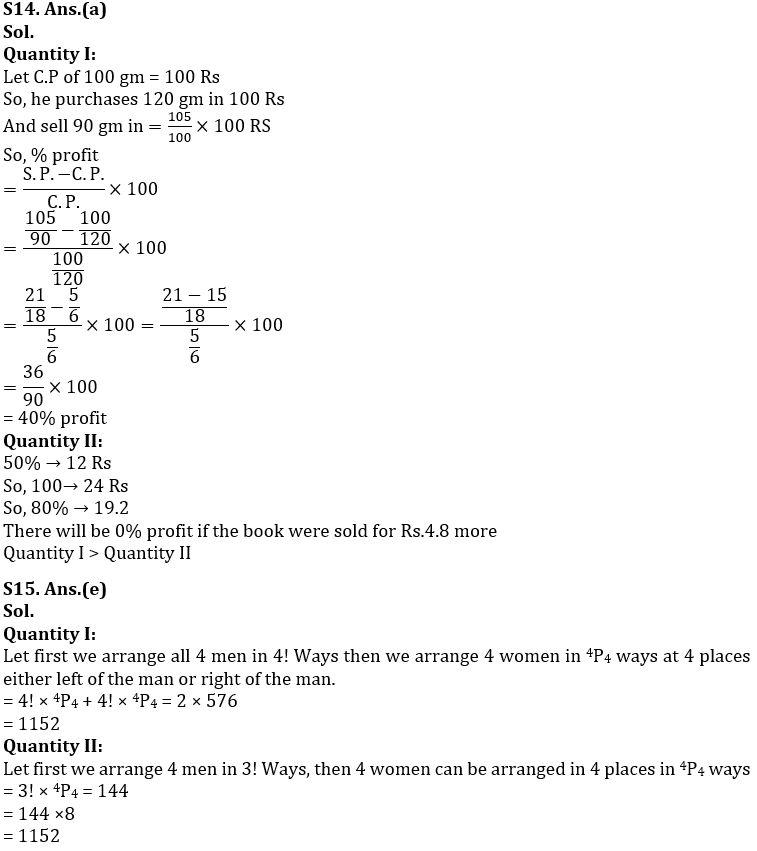#### Congratulations!